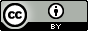# Bearden, A.P.; Schultz, T.W. Structure-Activity Relationships for Pimephales and Tetrahymena: a Mechanism of Action Approach. Environ. Toxicol. Chem. 1997, 16, 6, 1311–1317.

## QsarDB content

### Property pLC50: 96-h Fathead minnow toxicity as log(1/LC50) [log(L/mmol)]

##### 2: Nonpolar narcosis

Regression model (regression)

Open in:QDB Explorer QDB Predictor

Name Type n

R2

σ

Training training 12 0.859 0.306
##### 4: Polar narcosis

Regression model (regression)

Open in:QDB Explorer QDB Predictor

Name Type n

R2

σ

Training training 39 0.888 0.304
##### 6: Polar narcosis (phenols)

Regression model (regression)

Open in:QDB Explorer QDB Predictor

Name Type n

R2

σ

Training training 25 0.931 0.213
##### 8: Polar narcosis (anilines)

Regression model (regression)

Open in:QDB Explorer QDB Predictor

Name Type n

R2

σ

Training training 14 0.890 0.367
##### 10: Weak acid respiratory uncoupling

Regression model (regression)

Open in:QDB Explorer QDB Predictor

Name Type n

R2

σ

Training training 14 0.754 0.460
##### 12: Soft electrophilicity

Regression model (regression)

Open in:QDB Explorer QDB Predictor

Name Type n

R2

σ

Training training 17 0.196 0.995
##### 14: Proelectrophilicity

Regression model (regression)

Open in:QDB Explorer QDB Predictor

Name Type n

R2

σ

Training training 11 0.063 0.964
##### 17: Nonpolar narcosis (phenols)

Regression model (regression)

Open in:QDB Explorer QDB Predictor

Name Type n

R2

σ

Training training 25 0.924 0.233
##### 20: Soft electrophilicity

Regression model (regression)

Open in:QDB Explorer QDB Predictor

Name Type n

R2

σ

Training training 17 0.761 0.543
##### 22: Proelectrophilicity

Regression model (regression)

Open in:QDB Explorer QDB Predictor

Name Type n

R2

σ

Training training 11 0.836 0.403

### Property pIGC50: 2-day Tetrahymena toxicity as log(1/IGC50) [log(L/mmol)]

##### 1: Nonpolar narcosis

Regression model (regression)

Open in:QDB Explorer QDB Predictor

Name Type n

R2

σ

Training training 10 0.450 0.485
##### 3: Polar narcosis

Regression model (regression)

Open in:QDB Explorer QDB Predictor

Name Type n

R2

σ

Training training 38 0.387 0.685
##### 5: Polar narcosis (phenols)

Regression model (regression)

Open in:QDB Explorer QDB Predictor

Name Type n

R2

σ

Training training 25 0.380 0.710
##### 7: Polar narcosis (anilines)

Regression model (regression)

Open in:QDB Explorer QDB Predictor

Name Type n

R2

σ

Training training 13 0.698 0.453
##### 9: Weak acid respiratory uncoupling

Regression model (regression)

Open in:QDB Explorer QDB Predictor

Name Type n

R2

σ

Training training 13 0.691 0.450
##### 11: Soft electrophilicity

Regression model (regression)

Open in:QDB Explorer QDB Predictor

Name Type n

R2

σ

Training training 17 0.301 0.668
##### 13: Proelectrophilicity

Regression model (regression)

Open in:QDB Explorer QDB Predictor

Name Type n

R2

σ

Training training 11 0.568 0.529
##### 15: Nonpolar narcosis

Regression model (regression)

Open in:QDB Explorer QDB Predictor

Name Type n

R2

σ

Training training 38 0.389 0.672
##### 16: Nonpolar narcosis (phenols)

Regression model (regression)

Open in:QDB Explorer QDB Predictor

Name Type n

R2

σ

Training training 25 0.381 0.715
##### 18: Weak acid respiratory uncoupling

Regression model (regression)

Open in:QDB Explorer QDB Predictor

Name Type n

R2

σ

Training training 6 0.911 0.199
##### 19: Soft electrophilicity

Regression model (regression)

Open in:QDB Explorer QDB Predictor

Name Type n

R2

σ

Training training 17 0.670 0.461
##### 21: Proelectrophilicity

Regression model (regression)

Open in:QDB Explorer QDB Predictor

Name Type n

R2

σ

Training training 11 0.715 0.429

## Citing

When using this QDB archive, please cite (see details) it together with the original article:

• Ruusmann, V. Data for: Structure-Activity Relationships for Pimephales and Tetrahymena: a Mechanism of Action Approach. QsarDB repository, QDB.41. 2012. http://dx.doi.org/10.15152/QDB.41

• Bearden, A. P.; Schultz, T. W. Structure-Activity Relationships for Pimephales and Tetrahymena: a Mechanism of Action Approach. Environ. Toxicol. Chem. 1997, 16, 1311–1317. http://dx.doi.org/10.1002/etc.5620160629

 Title: Bearden, A.P.; Schultz, T.W. Structure-Activity Relationships for Pimephales and Tetrahymena: a Mechanism of Action Approach. Environ. Toxicol. Chem. 1997, 16, 6, 1311–1317. Abstract: The toxicity data of 74 chemicals tested in both the 96-h fathead minnow (Pimephales promelas) mortality assay and the 2-d Tetrahymena pyriformis (a protozoan) growth inhibition assay were evaluated using quantitative structure-activity relationships (QSARs). Each chemical was a priori assigned a mechanism of acute toxic action from either nonpolar narcosis, polar narcosis, weak acid respiratory uncoupling, soft electrophilicity, or proelectrophilicity. The polar narcotics were further split into a phenol group and an aniline group. The relationship between bioreactivity and the importance of penetration to the site of action in both systems was studied. Bioreactivity showed a trend to be inversely proportional to the value of the hydrophobicity term. The data were examined to investigate how different molecular descriptors modeled the mechanisms of action. Models were produced for nonpolar narcotics and anilines for both species with the 1-octanol/water partition coefficient (log Kow) alone. Soft electrophiles were best predicted by the average acceptor superdelocalizability (Snav), whereas proelectrophiles were modeled by log Kow and S(n)av. The weak acid uncouplers modeled with either log Kow or log Kow plus the ionization constant (pKa) for Pimephales and Tetrahymena, respectively. Phenols yielded predictive models using a either a combination of log Kow with S(n)av or lowest unoccupied molecular orbital, for fathead minnow and protozoan, respectively. URI: http://hdl.handle.net/10967/41 http://dx.doi.org/10.15152/QDB.41 Date: 2012-05-23
﻿

## Files in this item

Name Description Format Size View
i1552-8618-16-6-1311.qdb.zip n/a application/zip 25.83Kb View/OpenFiles associated with this item are distributed